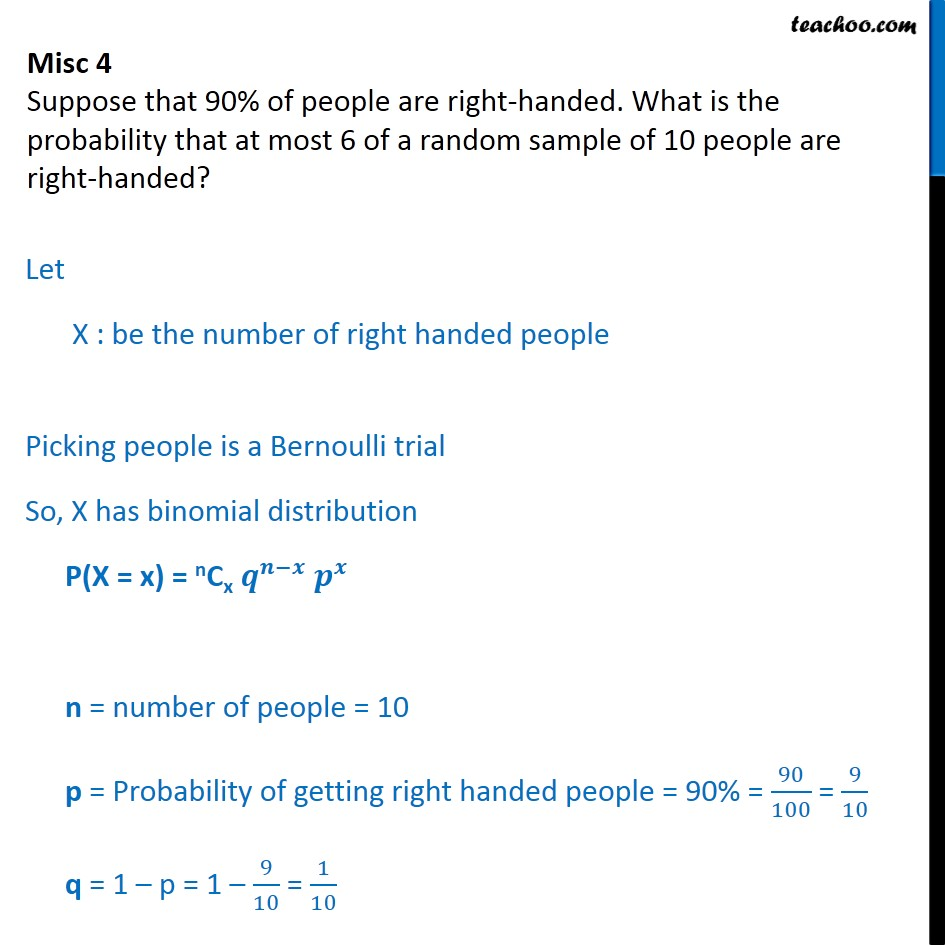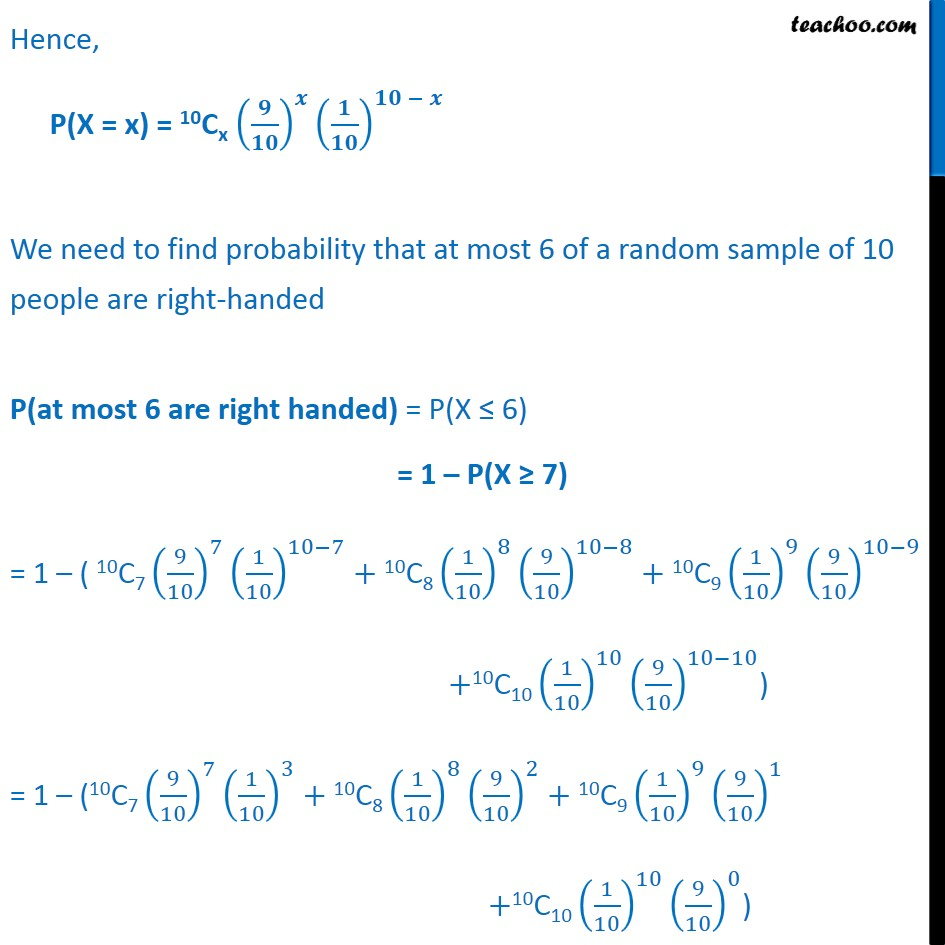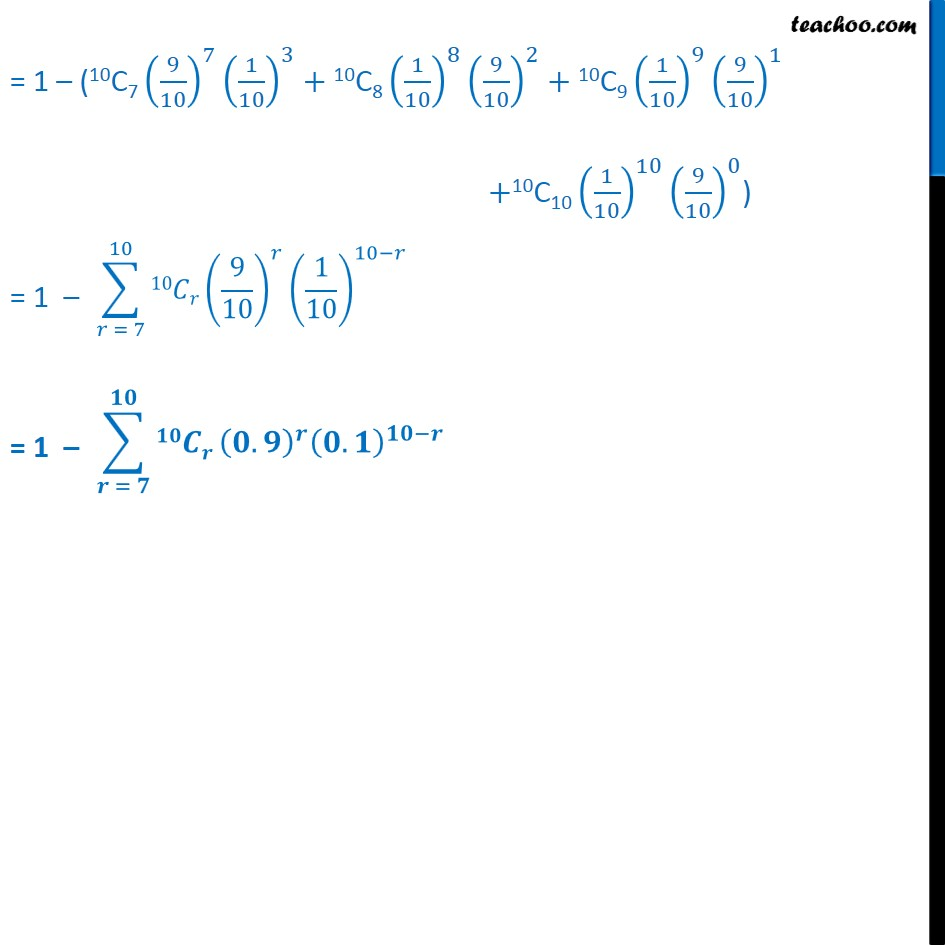Miscellaneous

Chapter 13 Class 12 Probability
Serial order wiseLearn in your speed, with individual attention - Teachoo Maths 1-on-1 Class

### Transcript

Misc 4 Suppose that 90% of people are right-handed. What is the probability that at most 6 of a random sample of 10 people are right-handed? Let X : be the number of right handed people Picking people is a Bernoulli trial So, X has binomial distribution P(X = x) = nCx 𝒒^(𝒏−𝒙) 𝒑^𝒙 n = number of people = 10 p = Probability of getting right handed people = 90% = 90/100 = 9/10 q = 1 – p = 1 – 9/10 = 1/10 Hence, P(X = x) = 10Cx (𝟗/𝟏𝟎)^𝒙 (𝟏/𝟏𝟎)^(𝟏𝟎 − 𝒙) We need to find probability that at most 6 of a random sample of 10 people are right-handed P(at most 6 are right handed) = P(X ≤ 6) = 1 – P(X ≥ 7) = 1 – ( "10C7" (9/10)^7 (1/10)^(10−7)+"10C8" (1/10)^8 (9/10)^(10−8)+"10C9" (1/10)^9 (9/10)^(10−9) +"10C10" (1/10)^10 (9/10)^(10−10)) = 1 – ("10C7" (9/10)^7 (1/10)^3+"10C8" (1/10)^8 (9/10)^2+"10C9" (1/10)^9 (9/10)^1+"10C10" (1/10)^10 (9/10)^0)∑_(𝒓 = 𝟕)^𝟏𝟎▒𝟏𝟎𝑪𝒓 (𝟎.𝟗)^𝒓 (𝟎.𝟏)^(𝟏𝟎−𝒓)